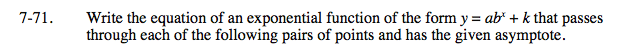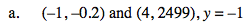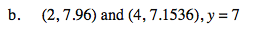Home > INT3 > Chapter 7 > Lesson 7.2.2 > Problem7-71

7-71.
1. Write the equation of an exponential function of the form y = abx + k that passes through each of the following pairs of points and has the given asymptote. Homework Help ✎

1. (–1, –0.2) and (4, 2499), y = –1

2. (2, 7.96) and (4, 7.1536), y = 7In this case,the horizontal asymptote is the vertical shift, or the k in the equation.Use the given points and asymptote to write and solve a system of two equations.

$-0.2 = ab^{-1} – 1\text{ }2499=ab^4-1$$y = 6(0.4)^x + 7$